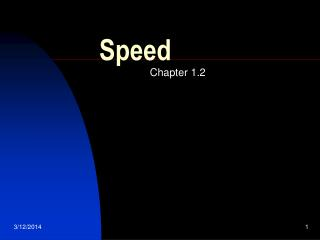DownloadDownload PresentationSpeed

# Speed

Download Presentation## Speed

- - - - - - - - - - - - - - - - - - - - - - - - - - - E N D - - - - - - - - - - - - - - - - - - - - - - - - - - -
##### Presentation Transcript

1. Speed Chapter 1.2

2. Introduction • An object’s position is measured from a reference point • To describe the position of an object, you can use distance and direction

3. To calculate speed, you need to know both distance and time • Objects that travel at different speeds move different distances in the same amount of time • Speed can be calculated by dividing the distance an object travels by the time it takes to cover the distance • The formula for finding speed is Speed = distance/time S=d/t

4. Standard Units • The standard units for speed in meters per second or kilometers per hour • In the English system speed is measured usually as miles per hour • One mile per hour equals .45 meters per second (m/s)

5. Average Speed • Your instantaneous speed , difficult to measure, is your moment-to-moment speed • Your average speed is the calculated measure of your speed over a distance.

6. Developing Graphing Skills • Speed is measured by the steepness, or, slope, of a line. • Slope is defined as the change in y-values divided by the change in x-values • A rising line, or positive slope, indicates that the distance an object travels from its starting point is increasing with time. • A horizontal line, or 0 slope, indicates that the speed is zero meters per second

7. Velocity • Velocity is a speed in a specific direction • Velocity is an example of a vector • A vector is a quantity that has both size and direction

8. What This Means • If you want to understand how an object (like a car, ball, person, or rocket) moves, you have to understand three things about what it means "to be moving." These three things "stick" to any object that moves, and are numbers that scientifically describe just how an object's motion is working. These three things are: • Position. This is precisely where an object is located. • Speed. Precisely how fast an object is moving. and..(the most difficult for most people)... • Acceleration. Precisely how fast an object's speed is changing.

9. Consider the motion of a Hot Wheels car down an incline, across a level and straight section of track, around a 180-degree curve, and finally along a final straight section of track. The car gains speed while moving down the incline - that is, it accelerates. Along the straight sections of track, the car slows down slightly (due to air resistance forces). Again the car could be described as having an acceleration. Finally, along the 180-degree curve, the car is changing its direction; once more the car is said to have an acceleration due to the change in the direction. Accelerating objects have a changing velocity - either due to a speed change (speeding up or slowing down) or a direction change.